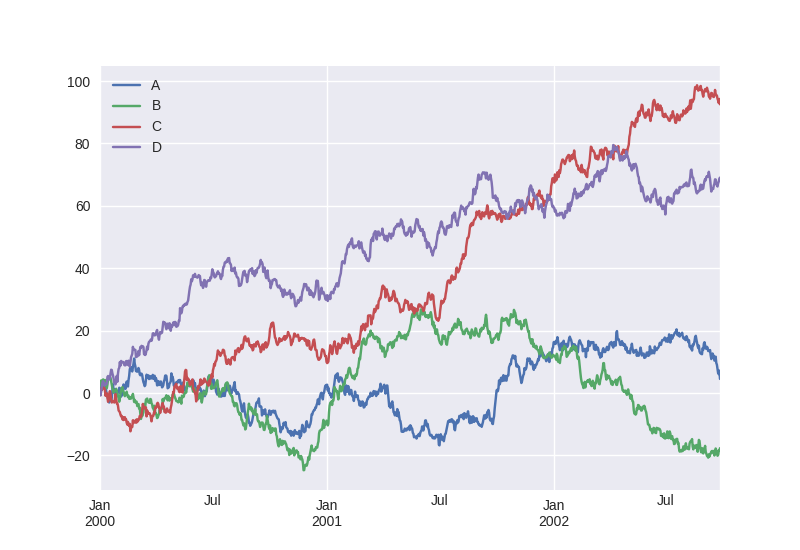# Welcome to Python¶

## Run some Python code!¶

To run the code below:

1. Click on the cell to select it.

2. Press `SHIFT+ENTER` on your keyboard or press the play button () in the toolbar above.

A full tutorial for using the notebook interface is available here.

```%matplotlib notebook

import pandas as pd
import numpy as np
import matplotlib

from matplotlib import pyplot as plt
import seaborn as sns

ts = pd.Series(np.random.randn(1000), index=pd.date_range('1/1/2000', periods=1000))
ts = ts.cumsum()

df = pd.DataFrame(np.random.randn(1000, 4), index=ts.index,
columns=['A', 'B', 'C', 'D'])
df = df.cumsum()
df.plot(); plt.legend(loc='best')
```
```<IPython.core.display.Javascript object>
``````<matplotlib.legend.Legend at 0x7f7f67afdf98>
```

Feel free to open new cells using the plus button (), or hitting shift-enter while this cell is selected.

Behind the scenes, the software that powers this is tmpnb, a Tornado application that spawns pre-built Docker containers and then uses the jupyter/configurable-http-proxy to put your notebook server on a unique path.